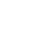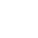LV. 30
GP 3k# 【心得】蛋池分析，我tm到底要存幾抽才夠? 2018/7/9更新

1.不歡迎帶節奏、陰謀論的人，要吵請去別的地方吵。
2.雖然這純粹是因為有興趣才開始算的，不過相信這對於各位在成本估計方面會很有幫助。
3.所有算法、程式碼都是透明公開的，歡迎各位找碴或幫忙優化

2018/7/8大更新:針對精準、擴充進行了詳細的分析，方便大家估一下成本和翻車率
2018/7/9小更新:針對日服機制進行了擴充初始S、精準up武器的分析

"如果"4星裝備，"真的"就是每次單抽的概率就是12.395%，那麼在沒有保底機制的情況下，四星裝備綜合概率為12.395%，可我們知道米忽悠是有保底，因此算上保底的話，四星裝備概率肯定大於12.395%!

1.沒有保底→出貨率為α(即清除保底的概率
2.觸發保底→出貨率為100%

"若非保底出貨概率為α，則期望次數為多少?"
 第1抽出貨的概率=α....第 i 抽出貨的概率=α*(1-α)^(i-1)....第9抽出貨的概率=α*(1-α)^8第10抽出貨的概率=(1-α)^9

1*α+2*α*(1-α)+3*α*(1-α)^2+4*α*(1-α)^3+5*α*(1-α)^4+6*α*(1-α)^5+7*α*(1-α)^6+8*α*(1-α)^7+9*α*(1-α)^8+10*1*(1-α)^9

●精準非保底出貨率:
1*α+2*α*(1-α)+3*α*(1-α)^2+4*α*(1-α)^3+5*α*(1-α)^4+6*α*(1-α)^5+7*α*(1-α)^6+8*α*(1-α)^7+9*α*(1-α)^8+10*1*(1-α)^9=1/12.395%

●擴充非保底出貨率:
1*α+2*α*(1-α)+3*α*(1-α)^2+4*α*(1-α)^3+5*α*(1-α)^4+6*α*(1-α)^5+7*α*(1-α)^6+8*α*(1-α)^7+9*α*(1-α)^8+10*1*(1-α)^9=1/15.000%

find_root(1*x+2*x*(1-x)+3*x*(1-x)^2+4*x*(1-x)^3+5*x*(1-x)^4+6*x*(1-x)^5+7*x*(1-x)^6+8*x*(1-x)^7+9*x*(1-x)^8+10*1*(1-x)^9-1/0.12395, x, 0, 1);

find_root(1*x+2*x*(1-x)+3*x*(1-x)^2+4*x*(1-x)^3+5*x*(1-x)^4+6*x*(1-x)^5+7*x*(1-x)^6+8*x*(1-x)^7+9*x*(1-x)^8+10*1*(1-x)^9-1/0.15000, x, 0, 1);

up武器:up聖痕:up外武器:up外聖痕=24:12:4:3

up武器..............1件，佔有比例20%
up聖痕..............3件，佔有比例30%
up外武器..........6件，佔有比例20%
up外聖痕........12件，佔有比例30%

 有50%概率up內有50%概率up外有40%概率出武器有60%概率出聖痕中up武器概率為20%中up聖痕概率為30%中"特定某個位置的up聖痕"概率為10%

 假設第K抽，保底已經累積 i 次，沒出貨(即沒清除保底)的機率為P(K,i)、非保底出貨的概率為α、確定出貨的前提下出up產物的概率β則我們有遞迴關係式初始值P(1,0)=α(1-β)P(1,1)=1-α1. i >0時，P(K, i )=(1-α)P(K-1, i -1)2. i =0時，P(K, i )=(1-β)P(K-1,9)+sum[ j ,0,8,α(1-β)P(K-1, j )]*α、β此兩常數隨池子類型、版本迭代更新可能會出現改動，請自行注意。顯而易見的，其實任意P(K,i)都是可以慢慢用遞迴算出來的。然後當我們有P(K,i)的詳細數據之後，那麼一口氣抽K次，出貨的概率也就能被算出來了假設一口氣抽K次，出貨的概率為P_get(K)，這個很簡單...所以ㄙㄨㄛ其實就是1減去所有抽不到的情況，而抽不到有幾中情況，分別為P(K,0) ~ P(K,9)所以P_get(K)=1-sum[ i ,0,9,P(K,i)]所以P(K,0)、*而日服的話，因為沒保底機制，所以情况就非常單純假設出貨的概率為α、一口氣抽K次，出貨的概率為P_get(K)1減去連續K抽都抽不到的概率，就是P_get(K)了所以日服的P_get(K)=1-(1-α)^K_get(K)

python3
CH

JP

●抽擴充
*非保底出A級以上角色的概率α為0.09427342057266558
*確定出A級以上角色，且角色為初始S的概率β為0.1

*大保底

●抽Up武器
*非保底出初始四星裝備的概率α0.04877033334098730
*確定出四星裝備，且裝備為up武器的概率β為:0.2
15發以內出貨的是歐洲人，
45發左右出貨的是亞洲人，
90發左右出貨的是非洲人。

*單兩者的單抽綜合概率其實是一樣的，不一樣的是概率分布

●抽Up聖痕*
*非保底出初始四星裝備的概率α0.04877033334098730
*確定出四星裝備，且裝備為up聖痕的概率β為0.3

*備註:

python3

face基於日前微軟官方表示 Internet Explorer 不再支援新的網路標準，可能無法使用新的應用程式來呈現網站內容，在瀏覽器支援度及網站安全性的雙重考量下，為了讓巴友們有更好的使用體驗，巴哈姆特即將於 2019年9月2日 停止支援 Internet Explorer 瀏覽器的頁面呈現和功能。

。Google Chrome（推薦）
。Mozilla Firefox
。Microsoft Edge（Windows10以上的作業系統版本才可使用）

face我們了解您不想看到廣告的心情⋯ 若您願意支持巴哈姆特永續經營，請將 gamer.com.tw 加入廣告阻擋工具的白名單中，謝謝 !【教學】